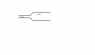# Volume flow rate and mass flow rate

• MNWO
In summary, the pipe has a gradually tapering section with a larger diameter at the end. The flow velocity is 1.26 m/s 3 in the larger diameter.f

#### MNWO

Hello there, so I have answered my question from fluid systems but the answer doesn't look right ;/ and I have no idea what have I done wrong. Can you please check and correct where it's wrong ? ;) here is the question:
A pipe contains a gradually tapering section in which its diameter decreases from 410mm to 260mm. The pipe contains an incompressible fluid of density 1000kgm- 3 and runs full. If the flow velocity is 2ms in the smaller diameter, determine the velocity in the larger diameter, the volume flow rate and the mass flow rate.

My calculations:
A1=260mm=0.26m
V1=2ms
A2=410mm=0.41m
V2=?
Flow rate (Q)=A1 x V1 which is equal to A2 x V2
0.26 x 2=0.52 m/s 3 (metres per second cubed)
V2= Q/A2 = 0.52/0.41
V2=1.26 m/s 3
mass flow rate (M)= Density x Q = 1000x 0.52
M=520 kg/s

I would really aprreciate any help :)

#### Attachments

•fluid system.png
676 bytes · Views: 662
Hello there, so I have answered my question from fluid systems but the answer doesn't look right ;/ and I have no idea what have I done wrong. Can you please check and correct where it's wrong ? ;) here is the question:
A pipe contains a gradually tapering section in which its diameter decreases from 410mm to 260mm. The pipe contains an incompressible fluid of density 1000kgm- 3 and runs full. If the flow velocity is 2ms in the smaller diameter, determine the velocity in the larger diameter, the volume flow rate and the mass flow rate.

My calculations:
A1=260mm=0.26m
V1=2ms
A2=410mm=0.41m
V2=?

Be careful here. You were given the diameters of the pipe, not the areas. How does the area of a pipe depend on its diameter?

Flow rate (Q)=A1 x V1 which is equal to A2 x V2
0.26 x 2=0.52 m/s 3 (metres per second cubed)
V2= Q/A2 = 0.52/0.41
V2=1.26 m/s 3
mass flow rate (M)= Density x Q = 1000x 0.52
M=520 kg/s

I would really aprreciate any help :)

See comment above.

•MNWO
Be careful here. You were given the diameters of the pipe, not the areas. How does the area of a pipe depend on its diameter?

See comment above.[/QUOT
Ah okay, i know what's wrong now. Thank you for that